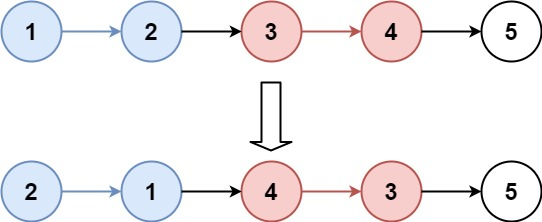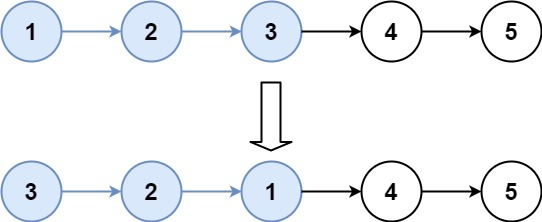# 25.reverse-nodes-in-k-group

## Statement

• 你可以设计一个只使用常数额外空间的算法来解决此问题吗？
• 你不能只是单纯的改变节点内部的值，而是需要实际进行节点交换。``````输入：head = [1,2,3,4,5], k = 2

````````````输入：head = [1,2,3,4,5], k = 3

``````

``````输入：head = [1,2,3,4,5], k = 1

``````

``````输入：head = , k = 1

``````

• 列表中节点的数量在范围 `sz`
• `1 <= sz <= 5000`
• `0 <= Node.val <= 1000`
• `1 <= k <= sz`

Given the `head` of a linked list, reverse the nodes of the list `k` at a time, and return the modified list.

`k` is a positive integer and is less than or equal to the length of the linked list. If the number of nodes is not a multiple of `k` then left-out nodes, in the end, should remain as it is.

You may not alter the values in the list's nodes, only nodes themselves may be changed.

Example 1:``````Input: head = [1,2,3,4,5], k = 2
Output: [2,1,4,3,5]
``````

Example 2:``````Input: head = [1,2,3,4,5], k = 3
Output: [3,2,1,4,5]
``````

Constraints:

• The number of nodes in the list is `n`.
• `1 <= k <= n <= 5000`
• `0 <= Node.val <= 1000`

Follow-up: Can you solve the problem in `O(1)` extra memory space?

## Solution

``````# Definition for singly-linked list.
# class ListNode:
#     def __init__(self, val=0, next=None):
#         self.val = val
#         self.next = next
from typing import Optional

class Solution:
def reverseKGroup(self, head: Optional[ListNode], k: int) -> Optional[ListNode]:
a = []

cur = ListNode()
res = cur

while len(a) >= k:
for i in range(k - 1, -1, -1):
cur.next = ListNode()
cur = cur.next
cur.val = a[i]
a = a[k:]

for x in a:
cur.next = ListNode()
cur = cur.next
cur.val = x

return res.next
``````